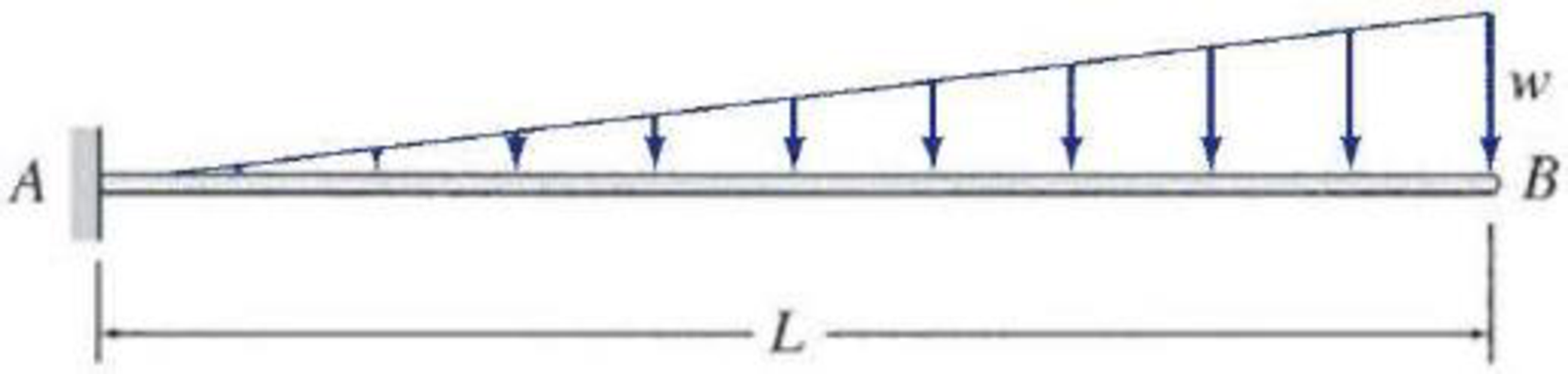# 6.1 through 6.6 Determine the equations for slope and deflection of the beam shown by the direct integration method. EI = constant. FIG. P6.5

#### Solutions

Chapter
Section
Chapter 6, Problem 5P
Textbook Problem
426 views

## 6.1 through 6.6 Determine the equations for slope and deflection of the beam shown by the direct integration method. EI = constant.FIG. P6.5To determine

Find the equations for slope and deflection of the beam using direct integration method.

### Explanation of Solution

Calculation:

Consider flexural rigidity EI of the beam is constant.

Draw the free body diagram of the beam as in Figure (1).

Refer Figure (1),

Consider upward force is positive and downward force is negative.

Consider clockwise moment is negative and counterclockwise moment is positive.

Since the end of the beam B is free, there will be no reaction.

Determine the support reaction at A using the relation;

V=0RA(12×w×L)=0RA=wL2

Determine the bending moment at A using the relation;

MA=0MA12×w×L×2L3=0MA=wL23

Show the reaction and moment as in Figure (2).

Draw the section at a distance x from support A as in Figure (3).

Write the equation for bending moment at x distance.

Mx=MA+RA(x)12×x×(wxL)×(13×x)=wL23+wL2x12x(wxL)(x3)=wL23+wL2xwx36L

Write the equation for MEI as follows:

d2ydx2=1EI(wL23+wL2xwx36L)        (1)

Write the equation for slope as follows:

Integrate Equation (1) with respect to x.

dydx=θ=1EI(wL23+wL2xwx36L)dx=1EI(wL23x+wL2(x22)w6L(x44))+C1        (2)

Write the equation for deflection as follows:

Integrate Equation (2) with respect to x.

y=[1EI(wL23x+wL2(x22)w6L(x44))+C1]dx=1EI[wL23(x22)+wL2(x36)w6L(x520)]+C1x+C2        (3)

Find the integration constants C1andC2:

Write the boundary conditions as follows:

A

### Still sussing out bartleby?

Check out a sample textbook solution.

See a sample solution

#### The Solution to Your Study Problems

Bartleby provides explanations to thousands of textbook problems written by our experts, many with advanced degrees!

Get Started

Find more solutions based on key concepts
For Problems 16.1 through 16.19, draw the top, the front, and the right-side orthographic views of the objects ...

Engineering Fundamentals: An Introduction to Engineering (MindTap Course List)

What is a code of ethics? Give an example.

Principles of Information Systems (MindTap Course List)

Explain the principles of adaptive cruise control.

Automotive Technology: A Systems Approach (MindTap Course List)

Why are sales discounts offered?

Cornerstones of Financial Accounting

You usually do not need to install web apps before you can run them.

Enhanced Discovering Computers 2017 (Shelly Cashman Series) (MindTap Course List)

What are the elements of a business impact analysis?

Principles of Information Security (MindTap Course List)

If your motherboard supports ECC DDR3 memory, can you substitute non-ECC DDR3 memory?

A+ Guide to Hardware (Standalone Book) (MindTap Course List)

Why is it important to not weld when everything is wet?

Welding: Principles and Applications (MindTap Course List)